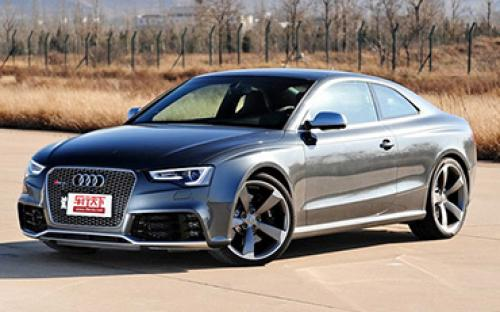### 奥迪RS 奥迪RS 59 种颜色可选2014款最低售价：109.80 万元起

4649(mm)1860(mm)1366(mm)##### 配置亮点：
• 胎压监测装置

• ISOFIX儿童座椅接口

• 车身稳定控制(ESC/ESP/DSC等)

• 电动天窗

• 定速巡航

• 后倒车雷达

• 真皮座椅

• GPS导航系统

• 氙气大灯

• 后视镜加热

• 提交

奥迪RS 奥迪RS 5 绕车实拍• 奥迪RS 奥迪RS 5 在售车型

排量 车型 厂商指导价 本地最低报价 购车工具
4.2L
RS 5 Coupe 特别版 7挡双离合
109.80万
109.80万

奥迪RS 奥迪RS 5 经销商

查看更多 >>

### 奥迪RS 奥迪RS 5 动力加速

奥迪RS 5 0-100公里加速时间分布在 4.5-4.9秒 属于 高性能级

动力级别 加速时间 车型
高性能级(2款)
4.5s
RS 5 Coupe 特别版
4.9s
RS 5 Cabriolet

奥迪RS 奥迪RS 5 视频

奥迪RS 奥迪RS 5 新闻资讯

# 设计小改动 奥迪发布RS 5 Coupe Sport

进口新车 超过5061次关注

日前，奥迪美国公司发布了一款RS5CoupeSport运动版车型，其在外观和内饰设计上相比普通版车型稍有不同。据悉，这款新车型将限量销售75台。

# 盘点德国队明星们的座驾 德系的垄断

评测 超过0次关注

八进决赛、四次捧杯、世界杯历史上总共打入224粒进球...无论是不是球迷，此前一个多月您一定是在无数有关世界杯的话题里度过，也会慢慢认识那么一批德国人，他们刚...

# RS 5 TDI概念车或量产 搭电动涡轮技术

新闻中心 超过5088次关注

奥迪RS5TDI概念车是为了纪念TDI发动机推出25周年而研发的，新车基于奥迪RS5Coupe车型打造而来。动力方面，奥迪RS5TDI概念车搭载的是3.0TDIV6双涡轮增压发动机，这台...

# 赛道试驾奥迪RS 5敞篷版 15秒的诱惑

车系文章 超过4859次关注

15秒能做什么？接一次吻，不能太深情。赏一口茶，不能太品味。那做什么？还好我们今天有奥迪RS5Cabriolet（敞篷版）助阵。15秒，你可以让自己与大自然零距离接触，...

# 奥迪RS 5敞篷版价格曝光

车系文章 超过3810次关注

从奥迪官方获悉，RS5Cabriolet敞篷版将会在10月底在中国地区上市，网络也曝光了其市场指导售价为128.8万元人民币。

# 实拍奥迪RS5 Coupe 挑战M3/C63 AMG

车系文章 超过5219次关注

“德国三驾马车”在全世界范围内拼得你死我活，而它们各自旗下的车型都有着明确的竞争指向性。唯独是高性能轿车这个细分区域，奥迪汽车在国内的发展步伐明显要比两...

# 奥迪RS 5展车到店 需提前预订 订金13万元

车系文章 超过3674次关注

近日，编辑从常州新奥汽车销售有限公司获悉，奥迪RS5展车到店，店内暂无现车销售，购车无优惠，需提前预订，订金13万元。

# 购奥迪RS 5享6万元优惠 有少量现车售

车系文章 超过3683次关注

近日，编辑从山东银座天尊汽车有限公司了解到，目前购奥迪RS5享6万元优惠，有少量现车售，提供试乘试驾车，有兴趣的朋友不妨到店或致电详询。

# 385马力/750N?m 奥迪RS5 TDI概念车官图

车系文章 超过3711次关注

日前，奥迪官方发布了RS5TDI概念车的官图，新车是为了纪念TDI发动机推出25周年而推出的。

猜你喜欢

﻿
• 快速找车
• 选择品牌
• 选择品牌
• A  奥迪
• A  阿斯顿·马丁
• A  阿尔法·罗密欧
• B  宝沃
• B  布加迪
• B  巴博斯
• B  保时捷
• B  宾利
• B  奔驰
• B  宝马
• B  本田
• B  别克
• B  标致
• B  比亚迪
• B  宝骏
• B  北汽制造
• B  北汽新能源
• B  北汽幻速
• B  北汽威旺
• B  北京汽车
• B  奔腾
• B  北汽绅宝
• C  长安
• C  长安商用
• C  长城
• C  昌河
• D  大众
• D  道奇
• D  DS
• D  东南
• D  东风风神
• D  东风风行
• D  东风小康
• D  东风风度
• D  东风
• F  福特
• F  丰田
• F  菲亚特
• F  法拉利
• F  福田
• F  福迪
• F  福汽启腾
• G  观致
• G  广汽传祺
• G  广汽吉奥
• G  GMC
• H  红旗
• H  汉腾汽车
• H  哈弗
• H  哈飞
• H  海格
• H  海马
• H  华颂
• H  黄海
• H  华泰
• H  恒天
• J  吉利汽车
• J  捷豹
• J  Jeep
• J  江淮
• J  江铃
• J  金杯
• J  九龙
• J  金旅
• K  凯翼
• K  凯迪拉克
• K  克莱斯勒
• K  科尼塞克
• K  卡威
• K  开瑞
• L  路虎
• L  林肯
• L  劳斯莱斯
• L  兰博基尼
• L  雷克萨斯
• L  铃木
• L  雷诺
• L  理念
• L  力帆
• L  莲花汽车
• L  猎豹
• L  路特斯
• L  陆风
• M  马自达
• M  MG
• M  MINI
• M  玛莎拉蒂
• M  摩根
• M  迈凯轮
• N  纳智捷
• O  欧宝
• O  讴歌
• O  欧朗
• Q  奇瑞
• Q  起亚
• Q  启辰
• R  日产
• R  荣威
• R  瑞麒
• S  三菱
• S  斯威汽车
• S  萨博
• S  smart
• S  斯柯达
• S  斯巴鲁
• S  思铭
• S  双龙
• S  上汽大通
• S  双环
• T  特斯拉
• T  腾势
• W  沃尔沃
• W  五菱汽车
• W  五十铃
• W  威兹曼
• W  威麟
• X  现代
• X  雪佛兰
• X  雪铁龙
• X  西雅特
• Y  一汽
• Y  英菲尼迪
• Y  英致
• Y  依维柯
• Y  野马汽车
• Y  永源
• Z  众泰
• Z  中华
• Z  中兴
• Z  知豆
• 选择车系
• 选择车系
• 车型对比
• 选择品牌
• 选择品牌
• A  奥迪
• A  阿斯顿·马丁
• A  阿尔法·罗密欧
• B  宝沃
• B  布加迪
• B  巴博斯
• B  保时捷
• B  宾利
• B  奔驰
• B  宝马
• B  本田
• B  别克
• B  标致
• B  比亚迪
• B  宝骏
• B  北汽制造
• B  北汽新能源
• B  北汽幻速
• B  北汽威旺
• B  北京汽车
• B  奔腾
• B  北汽绅宝
• C  长安
• C  长安商用
• C  长城
• C  昌河
• D  大众
• D  道奇
• D  DS
• D  东南
• D  东风风神
• D  东风风行
• D  东风小康
• D  东风风度
• D  东风
• F  福特
• F  丰田
• F  菲亚特
• F  法拉利
• F  福田
• F  福迪
• F  福汽启腾
• G  观致
• G  广汽传祺
• G  广汽吉奥
• G  GMC
• H  红旗
• H  汉腾汽车
• H  哈弗
• H  哈飞
• H  海格
• H  海马
• H  华颂
• H  黄海
• H  华泰
• H  恒天
• J  吉利汽车
• J  捷豹
• J  Jeep
• J  江淮
• J  江铃
• J  金杯
• J  九龙
• J  金旅
• K  凯翼
• K  凯迪拉克
• K  克莱斯勒
• K  科尼塞克
• K  卡威
• K  开瑞
• L  路虎
• L  林肯
• L  劳斯莱斯
• L  兰博基尼
• L  雷克萨斯
• L  铃木
• L  雷诺
• L  理念
• L  力帆
• L  莲花汽车
• L  猎豹
• L  路特斯
• L  陆风
• M  马自达
• M  MG
• M  MINI
• M  玛莎拉蒂
• M  摩根
• M  迈凯轮
• N  纳智捷
• O  欧宝
• O  讴歌
• O  欧朗
• Q  奇瑞
• Q  起亚
• Q  启辰
• R  日产
• R  荣威
• R  瑞麒
• S  三菱
• S  斯威汽车
• S  萨博
• S  smart
• S  斯柯达
• S  斯巴鲁
• S  思铭
• S  双龙
• S  上汽大通
• S  双环
• T  特斯拉
• T  腾势
• W  沃尔沃
• W  五菱汽车
• W  五十铃
• W  威兹曼
• W  威麟
• X  现代
• X  雪佛兰
• X  雪铁龙
• X  西雅特
• Y  一汽
• Y  英菲尼迪
• Y  英致
• Y  依维柯
• Y  野马汽车
• Y  永源
• Z  众泰
• Z  中华
• Z  中兴
• Z  知豆
• 选择车系
• 选择车系
• 选择车型
• 选择车型
• 意见反馈